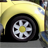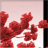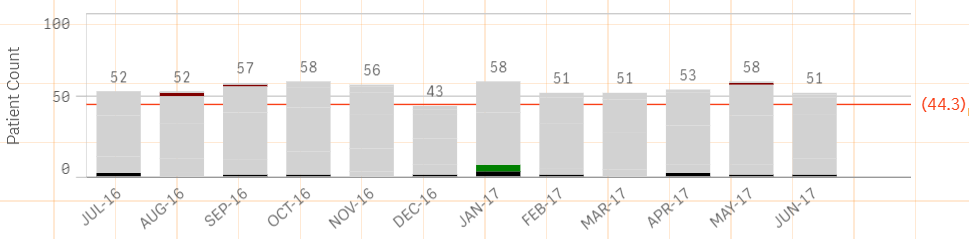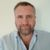# New to Qlik Sense

If you’re new to Qlik Sense, start with this Discussion Board and get up-to-speed quickly.

Announcements
Skip the ticket, Chat with Qlik Support instead for instant assistance.
cancel
Showing results for
Did you mean:Creator

## Color consistency b/w Bar chart vs Pie Chart

Hi ,

I have a requirement like below.

I want to show the same color for Pie chart as well as bar chart.

Dimension for  Bar chart --  I used 2 dimensions (Month Name (Jan-17).... Dec-17 ,Days)

Days Dimension -- I have used

if([xyz] <=1, 'Below 1 day'

',if([xyz] <=3,  'Below 2-3 day'

',if([xyz] <=7,'Below 3-7 day'

',if([xyz] <=17,'Below  8-17 day'

',if([xyz] <=30, 'Below 18-30 day'

,above 30 days

)'))))

Measure - Used    Count([xyz])+ 0 * Count([xyz])

Same thing for pie chart also

I used color by expression in both charts

if([xyz] <=1, Yellow()

',if([xyz] <=3, blue()

',if([xyz] <=7, Green()

',if([xyz] <=17, brown()

',if([xyz] <=30, red()

,Red()

)'))))

But still it's not coming correctly. Kindly help me on this

1 Solution

Accepted SolutionsMVP

In essence, use the same set analysis that you use in your expression here

Only({<SetAnalysisFromYourExpression>}

if([xyz] <=1, Yellow()

',if([xyz] <=3, blue()

',if([xyz] <=7, Green()

',if([xyz] <=17, brown()

',if([xyz] <=30, red()

,Red()

)'))))

)

12 RepliesSpecialist

I think you have to re write your if condition like you have to mention condition like,

if([xyz]<=1,yellow(),if([xyz]>1 and [xyz]<=3,blue(),if([xyz]>3 and [xyz]<=7,green())))

because in above condition there is confusion you are mentioning different colors for <=1,<=3 and so on,e.g. if your value is -1 then statement is true for both the conditionMVP

Would you be able to share a sample to show the issue?Creator
Author

I have tied this too. but no luck

IF([Length of Stay] <= 1 ,  rgb(0,0,0),

If([Length of Stay] >1 AND  [Length of Stay] <=3,  Green(),

if(  [Length of Stay] >3  AND  [Length of Stay] <= 7 , Yellow(),

if(  [Length of Stay] >7  AND  [Length of Stay] <=17 , CYAN(),

if( [Length of Stay] >17  AND  [Length of Stay] <=30 , Red ()

, Magenta()

)))))Specialist

Days dimension you have calculated on chart or at script level.

because if you have calculated at script then you can try condition like,if([xyz]='Below 1 day',blue(),if([xyz]='Below 2-3 day',yellow())) and so on.Creator
Author

Hi Sunny,

Here, I can't see the different colors for different categoryMVP

Try this... I think the issue is that you might be making selections which might be causing this. I have used {1} to ignore all selections for your color expression

Only({1}

if([xyz] <=1, Yellow()

',if([xyz] <=3, blue()

',if([xyz] <=7, Green()

',if([xyz] <=17, brown()

',if([xyz] <=30, red()

,Red()

)'))))

)Partner - Master II

The best way to manage colour consistently across your apps is to make your standard dimensions and measures master items and then use the built in feature to colour particular values.

Then when you create a chart of whatever type using those master items you can set it to use libray colours and your chart will use those master colours.

Regards

AndyCreator
Author

It's working partially.

Eg :

I am able to see the different color for different category.

ButIf select year = 2017 . i want to display only 12 months.

But in our expression.it pull all the months for all years rather than particular yearMVP

Do you have a set analysis that you use in your main expression to see 12 months?Tags
Community Browser